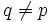# Analogue of Thompson transitivity theorem fails for groups in which not every p-local subgroup is p-constrained

It is possible to choose a finite group$G$ and a prime number$p$ such that$G$ is not a group in which every p-local subgroup is p-constrained, and such that:
There is a subgroup$A$ that is maximal among abelian normal subgroups in some$p$-Sylow subgroup of$G$ such that the rank of$A$ is at least three, and there is a prime$q \ne p$ such that$C_G(A)$ is not transitive on the set of maximal$A$-invariant$q$-subgroups.